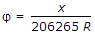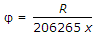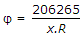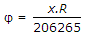# Civil Engineering - Surveying - Discussion

### Discussion :: Surveying - Section 2 (Q.No.18)

18.

Let angular value of one graduation of a tube of length x be φ seconds and R be the radius of its internal curved surface, then

 [A].[B].[C].[D]..

Explanation:

No answer description available for this question.

 Bestengineer said: (Sep 2, 2016) How? Explain.

 Rajendra said: (Jan 30, 2017) It should be( x/R ) * 206265.

 Abhishek Anand said: (Feb 11, 2017) Yeah,angle= (arc/radius) radians. = (d/r) * 206265 sec. = (206265d/r). (1rad=206265sec).

 Er Meghanada (Bhadrak) said: (Nov 17, 2017) x = Angular value of one graduation of tube of length. φ = Second. R = Radius. D = 206265 sec. (x/r)*d = (x/r)*206265. φ = x/206265.

 Amjad Ali said: (Feb 15, 2020) None of these. Sensitivity= x*206265/R.

 Raju Sarkar said: (Jun 9, 2020) Yes, it should be (x/R)206265.

 Shiva said: (Jan 30, 2021) Thanks @ Er.Meghanada.

 Mesbah Ullah said: (Mar 6, 2021) Arc = φ *R......... φ in rad. φ rad = Arc/R. φ = Arc/(R*rad) = x/(R*206265).

 Mjm Gcek said: (Aug 13, 2021) Yes, you are correct @Rahendra.